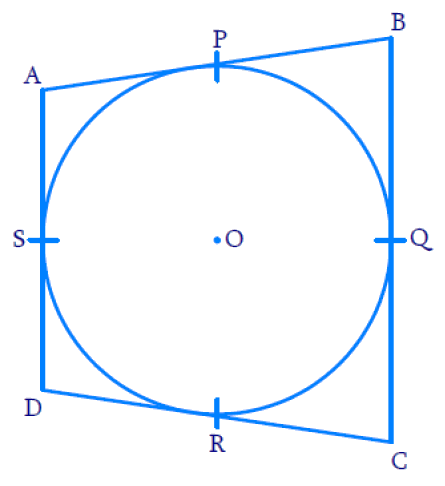# Ex.10.2 Q11 Circles Solution - NCERT Maths Class 10

Go back to  'Ex.10.2'

## Question

Prove that the parallelogram circumscribing a circle is a rhombus.

Video Solution
Circles
Ex 10.2 | Question 11

## Text Solution

To prove:

The parallelogram circumscribing a circle is a rhombus

Reasoning:$${ABCD}$$ is a parallelogram. Therefore, opposite sides are equal.

\begin{align}\therefore {AB} & = {CD} \\ {BC} & = {AD} \end{align}

According to Theorem 10.2 : The lengths of tangents drawn from an external point to a circle are equal.

Steps :

Therefore,

$$BP = BQ$$ (Tangents from point $$B$$)…… ($$1$$)

$$CR = CQ$$ (Tangents from point $$C$$)…… ($$2$$)

$$DR = DS$$ (Tangents from point $$D$$)…… ($$3$$)

$$AP = AS$$ (Tangents from point $$A$$)……. ($$4$$)

Adding $$(1) + (2) + (3) + (4)$$

\begin{align} & BP+CR+DR+AP \\ & =BQ+CQ+DS+AS \\ \end{align}
On re-grouping,

\begin{align} \left[ \begin{array} & BP+AP+ \\ CR+DR \\ \end{array} \right]&=\left[ \begin{array} & BQ+CQ+ \\ DS+AS \\ \end{array} \right] \\ AB+CD&=BC+AD \\ \end{align}

Substitute $${CD = AB}$$ and $${AD = BC}$$ since $${ABCD}$$ is a parallelogram, then

\begin{align}{ A B + A B} & = {B C + B C} \\ {2 A B} & ={ 2 B C} \\ {A B} & = {BC} \end{align}

$$\therefore {AB=BC=CD=DA}$$

This implies that all the four sides are equal.

Therefore, the parallelogram circumscribing a circle is a rhombus.

Learn from the best math teachers and top your exams

• Live one on one classroom and doubt clearing
• Practice worksheets in and after class for conceptual clarity
• Personalized curriculum to keep up with school